Next: Flow Around a Submerged Up: Axisymmetric Incompressible Inviscid Flow Previous: Conformal Maps

# Flow Around a Submerged Oblate Spheroid

Consider the conformal map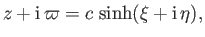(7.115)

where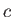is real and positive. It follows that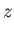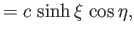(7.116)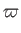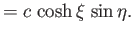(7.117)

Let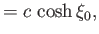(7.118)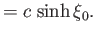(7.119)

Thus, in the meridian plane, the curvecorresponds to the ellipse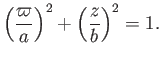(7.120)

We conclude that the surfaceis an oblate spheroid (i.e., the three-dimensional surface obtained by rotating an ellipse about a minor axis) of major radiusand minor radius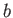. The constraints (7.87) and (7.90) yield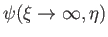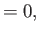(7.121)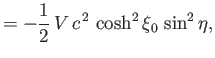(7.122)

respectively. Setting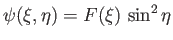, and substituting into the governing equation, (7.86), we obtain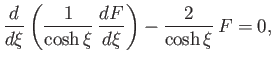(7.123)

which can be rearranged to give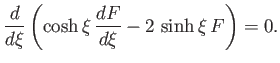(7.124)

On integration, we get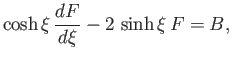(7.125)

which can be rearranged to give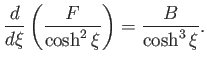(7.126)

It follows that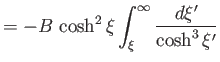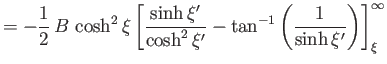(7.127)

where use has been made of the constraint (7.121). Let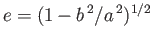be the eccentricity of the spheroid. Thus,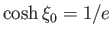,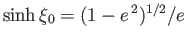,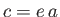, and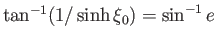. The constraint (7.122) yields(7.128)

or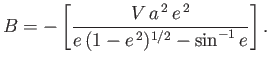(7.129)

Hence,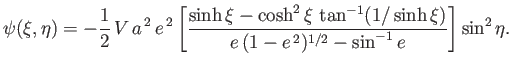(7.130)

Finally, from Equation (7.84),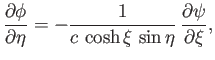(7.131)

which can be integrated to give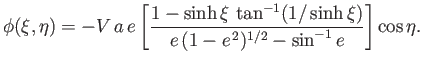(7.132)

It is easily demonstrated that(7.133)

and(7.134)

Thus,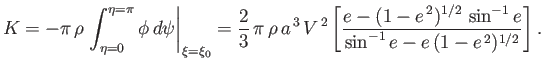(7.135)

It follows that the added mass of the spheroid is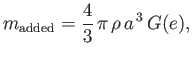(7.136)

where(7.137)

is a monotonic function that varies between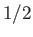whenandwhen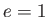.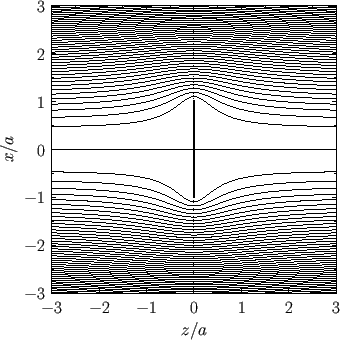In the limit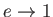, our spheroid asymptotes to a thin disk of radiusmoving through the fluid in the direction perpendicular to its plane. Expressions (7.130), (7.132), and (7.136) yield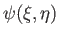(7.138)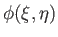(7.139)

and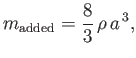(7.140)

respectively. Here,and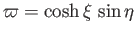. It follows that, in the instantaneous rest frame of the disk,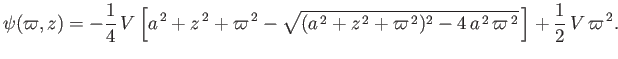(7.141)

This flow pattern, which corresponds to that of a thin disk placed in a uniform flow perpendicular to its plane, is visualized in Figure 7.6.Next: Flow Around a Submerged Up: Axisymmetric Incompressible Inviscid Flow Previous: Conformal Maps
Richard Fitzpatrick 2016-03-31